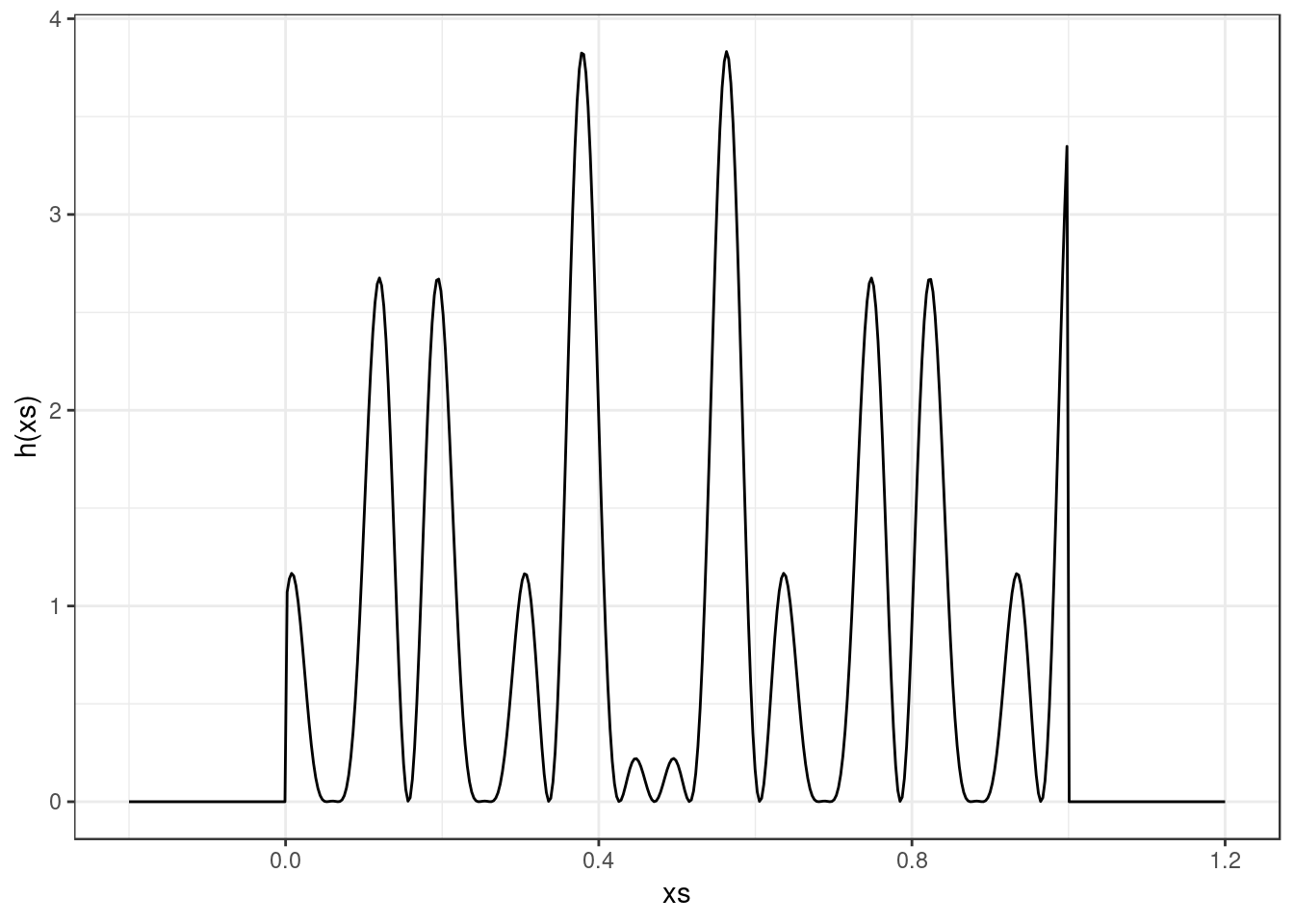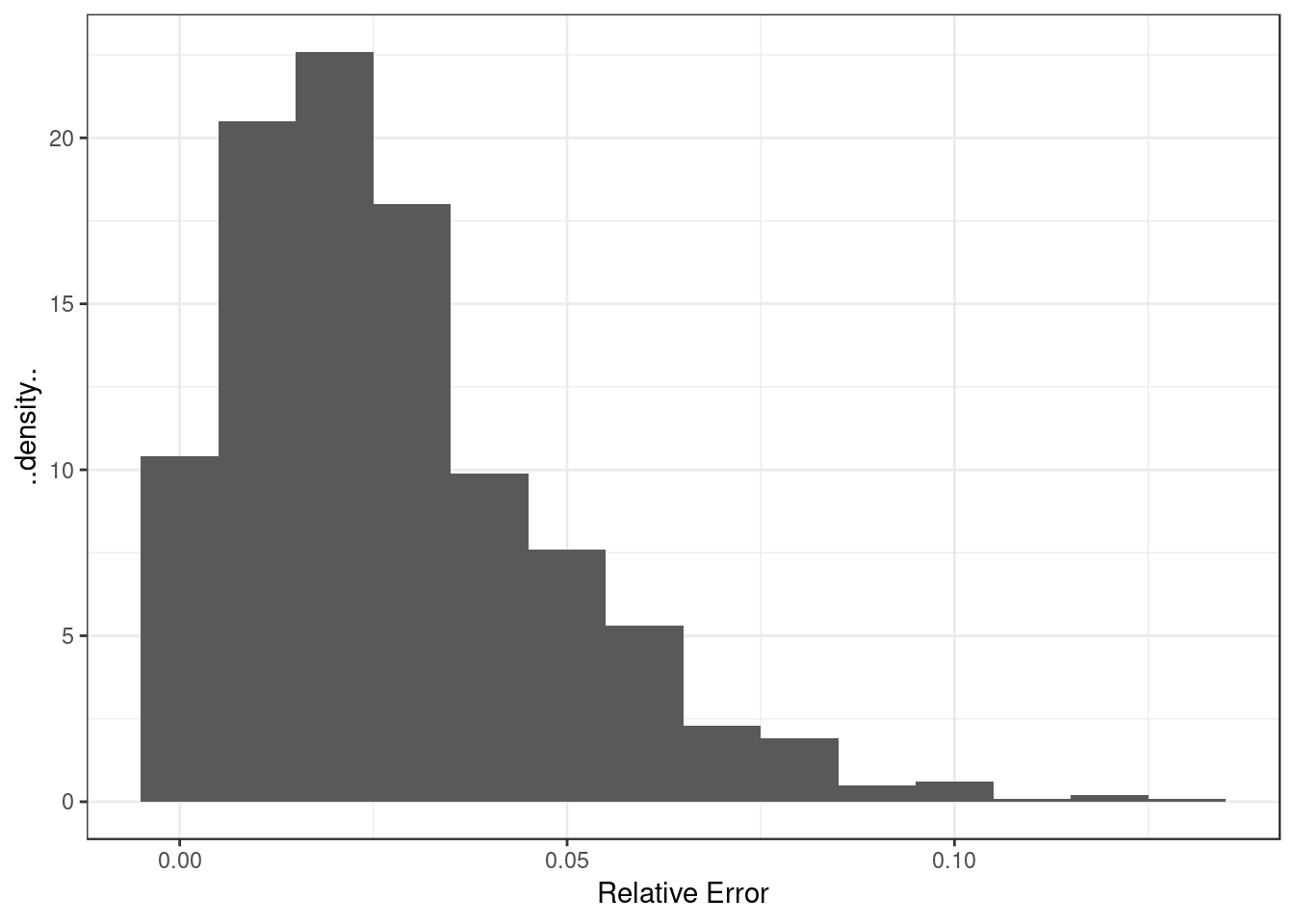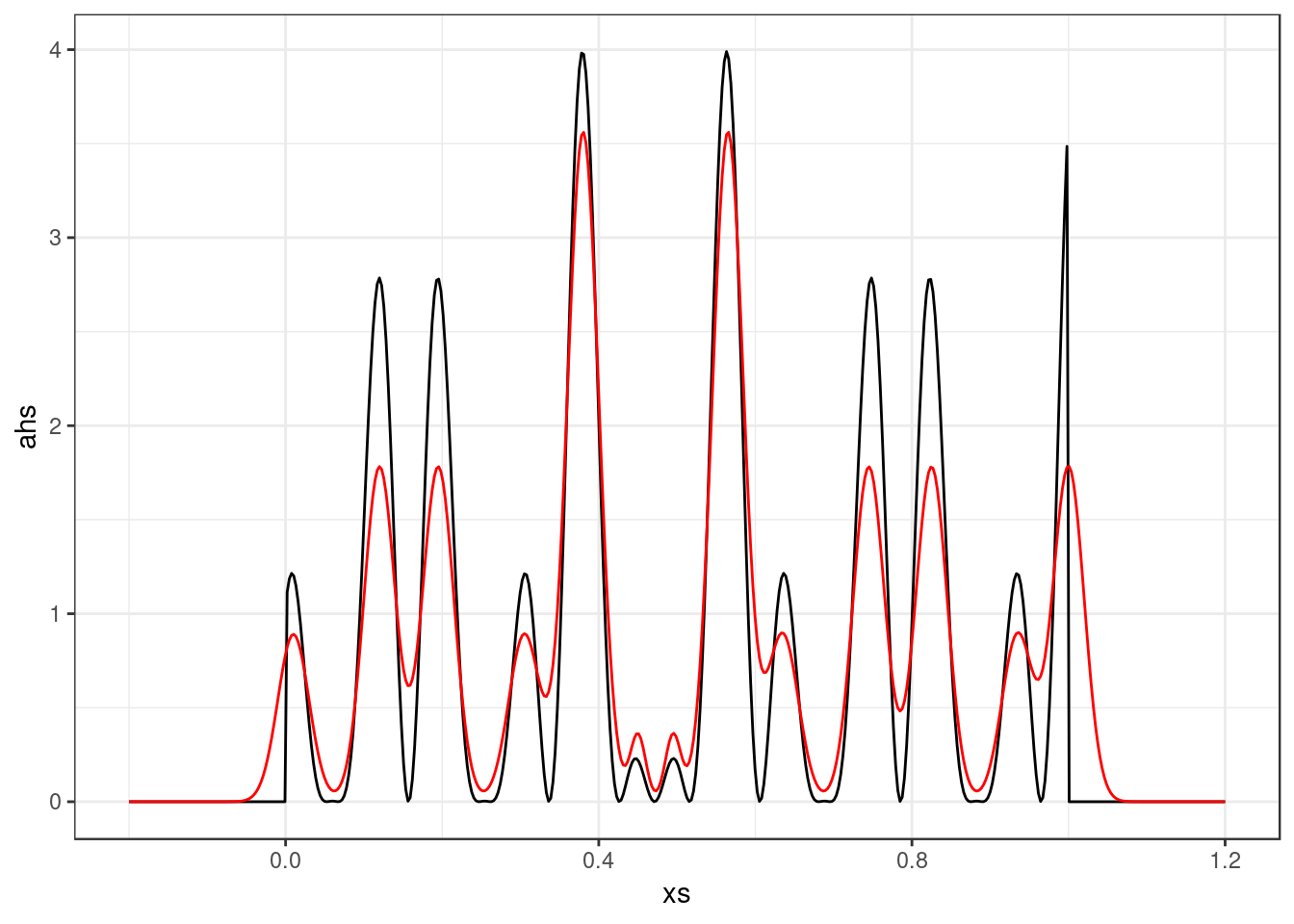## Importance sampling

Note: the function $$h$$ was to be defined as the of the function given in the exercise. As it stands, the question would give a very small result since it has approximately equal positive and negative parts. See below for a solution to the square.

First we define the function $h(x) = \left[ \cos(50x) + \sin(20x) \right]^2.$

h <- function(x) {
z <- (x > 0) * (x < 1) * (cos(50 * x) + sin(20 * x))
z^2
}

qplot(x = xs, y = h(xs), geom = "line")$$h$$ can be integrated analytically. Expanding $h(x) = \cos(50x)^2 + 2 \cos(50x) \sin(20x) + \sin(20x)^2,$ the antiderivative in $$[0, 1]$$ is $H(x) = \frac{x}{2} + \frac{\sin(100x)}{200} + 2 \left[ \frac{\cos(30x)}{60} - \frac{\cos(70x)}{140} \right] + \frac{x}{2} - \frac{\sin(40x)}{80}$ and integrated over $$[0, 1]$$ gives

H <- function(x) {
x/2 + sin(100 * x)/200 + 2 * (cos(30 * x)/60 - cos(70 * x)/140) + x/2 -
sin(40 * x)/80
}
trueValue <- H(1) - H(0)
cat("The true value of I is", trueValue)
## The true value of I is 0.9652009

### Uniform Proposal

First, rejection sampling with uniform proposal amounts here to crude Monte Carlo here, as the integral is with respect to the uniform measure on $$[0,1]$$.

numRepeats <- 1000
numPoints <- 1000
mcErrors <- rep(NA, numRepeats)
for (i in 1:numRepeats) {
u <- runif(n = numPoints)
weights <- h(u)
mcErrors[i] <- abs(mean(weights) - trueValue)/trueValue
}

qplot(x = mcErrors, y = ..density.., geom = "histogram", binwidth = 0.01) +
xlab("Relative Error")### Gaussian Mixture Proposal

From our plot of $$h$$ we realize it is multimodal. We expect that we should be able to reduce the variance of the Monte Carlo estimator by using importance sampling (IS) with a Gaussian mixture proposal. We roughly place some mixture components by eye here, but feel free to try to automate this procedure using an optimizer, for example.

numComponents <- 13
omega <- c(1, 2, 2, 1, 4, 0.2, 0.2, 4, 1, 2, 2, 1, 2)
omega <- omega/sum(omega)
mu <- c(0.01, 0.12, 0.195, 0.305, 0.38, 0.45, 0.495, 0.565, 0.635, 0.745, 0.825,
0.935, 1)
sigma <- c(0.02, 0.02, 0.02, 0.02, 0.02, 0.01, 0.01, 0.02, 0.02, 0.02, 0.02,
0.02, 0.02)

# Use a builtin library for sampling from normal mixtures.
library(nor1mix)
mixture <- norMix(m = mu, sigma = sigma, w = omega)

Let’s visually compare the target function with our importance sampling density.

normalized_hs <- abs(h(xs))/sum(abs(h(xs))) * 500/1.4

df <- data.frame(xs = xs, hs = abs(h(xs)), ahs = normalized_hs, qs = dnorMix(xs,
mixture))
ggplot(df) + geom_line(aes(x = xs, y = ahs), colour = "black") + geom_line(aes(x = xs,
y = qs), colour = "red")qplot(x = xs, y = h(xs)/dnorMix(xs, mixture), col = "blue")